Next: Continuous Hidden Variables CEM Up: From EM to CEM Previous: From EM to CEM

## Discrete Hidden Variables CEM

We shall now concentrate our attention on the case of discrete m(i.e. m takes on a discrete integer value from between [1,M]) which can be considered as an index into the pdf to select each of the individual simpler components. The continuous missing data case will be considered later.

The EM algorithm, in essence, uses Jensen's inequality to find a lower bound to the above quantity by taking advantage of the linear superposition qualities of the individual probability models. The log likelihood (the function being maximized) is manipulated using this simple bounding technique in Equation 7.4  . Jensen's inequality lower bounds the logarithm of the sum to make it easier to handle. The fundamental result is that the logarithm is applied to each simple model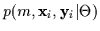individually instead of applying to the whole sum. It is then straightforward to compute the derivatives with respect to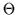and set those equal to zero to do the maximization.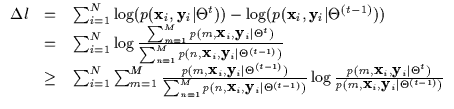(7.4)

Let us now consider the case when we are estimating a conditional density . Conditioning the discrete mixture model in Equation 7.3 results in Equation 7.5. This is a conditional density with missing data m which has been re-written as a joint density with missing data m over a marginal density with missing data m. We shall show that this missing data problem can not be solved using the standard EM approach.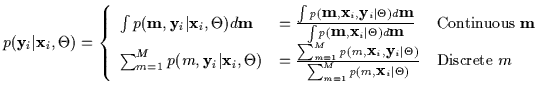(7.5)

The conditional log-likelihood utilizes this density model and is evaluated as in Equation 7.6. This is also an incremental expression (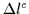) indicating that we are maximizing the improvement the model undergoes with each iteration (versus directly maximizing the model itself).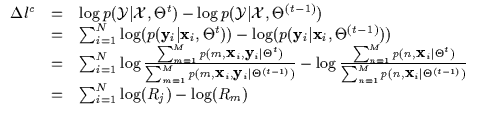(7.6)

Note that the above expression is the difference between the logarithms of two ratios (Rj and Rm). Rj is the ratio of the new joint over old joint and Rm is the ratio of the new marginal over the old marginal. In maximum likelihood however, only the first term is present (Rj). We could obtain a lower bound for it using Jensen's inequality. Since the log function is concave, Jensen will lower bound the log of a sum or expectation. However, in the maximum conditional likelihood situation, we subtract the second term (the ratio of marginals). Jensen can provide a lower bound for this second term however it is being subtracted. Since subtracting the second terms indicates that we are using the negative of the lower bound it instead acts as an upper bound. Thus, we will not be bounding conditional likelihood if we apply Jensen's inequality to the second term and can no longer guarantee convergence. Thus, let us refrain and only apply it to the first term (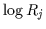) as in Equation 7.7.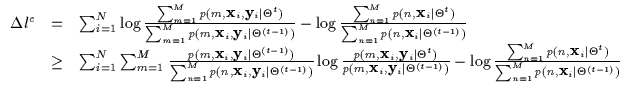(7.7)

As a side note we can see that the conditional density is tricky because it is the joint divided by the marginal (over x). For a mixture model, the numerator (the joint) is a linear combination of multiple models. However, the marginal is also a linear combination of models and it resides in the denominator. Thus, linear superposition occurring in joint mixture models does not hold when they are conditioned and things become more unmanageable. In fact, it seems that we simply can not pull the summation outside of the logarithm in the above expression to end up with an elegant maximization step.

At this point, if we were only considering EM approaches, we would typically resort to a Generalized Expectation Maximization approach (GEM)  which involves some gradient ascent techniques to make up for the inapplicability of EM. For instance, the Iteratively Re-Weighted Least Squares algorithm does such an operation in the Mixtures of Experts   architecture. The estimation uses EM on a piece of the problem and then resorts to a gradient ascent approach in an inner loop which can not be posed as a typical EM iteration. This is still better than performing the whole conditional likelihood maximization completely with gradient ascent (as proposed by Bishop ). This is due to the extra convergence properties the EM-bound provides on top of the inner gradient ascent steps. However, it is not necessary to, dare we say, throw in the towel and resort to gradient ascent quite yet if more bounding techniques (i.e. other than EM and Jensen) can be first investigated. We shy away from the gradient ascent technique since it is often plagued with ad hoc step size estimation, slow non-monotonic convergence, and susceptibility to local maxima.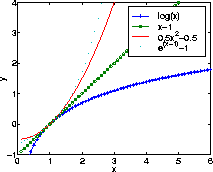Recall earlier that we discussed the existence of numerous bounds for upper and lower bounding. It is important, in addition, that the bound chosen makes contact with the desired function at the current operating point. In the above case, the so-called current operating is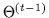, the previous state of the model we are optimizing. When we are at our current operating point (i.e.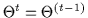), the ratio of the marginals between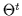andmust equal 1. Thus, the logarithm of the Rm ratio in the expression above can be upper bounded and thus the negative of the logarithm will be lower bounded. Many functions can used to upper bound the logarithm as shown again in Figure  7.1. The choices of the bounds include the following functions: x-1,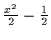, and ex-1. We choose the tightest convex upper bound on the log which is x-1. It also seems the simplest to manipulate. This manipulation removes the cumbersome logarithm of a summation and essentially decouples the sub-models that are added inside. We end up with Equation 7.8 which is much easier to manipulate. In fact, for our purposes, this expression will act as the analog of the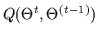function for the EM algorithm.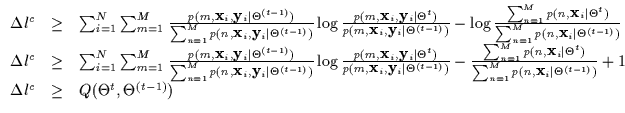(7.8)

At this point, we re-write a conditioned mixture model expression without any joint density models in Equation 7.9. This is similar to the Mixture of Experts notation  where many conditional models (called experts) are gated by marginal densities. This decomposition introduces an important flexibility in the conditional density: the marginal densities or gates need not integrate to one and can merely be arbitrary positive functions f() instead of probability densities p(). This generalization does not violate the form of the conditional density in any way and only indicates that the gates need not be pdfs and need not integrate to 1 or any finite number. Thus, the gates can diverge, have discontinuities, grow towards infinity and so on (as long as they remain positive) and still maintain a legal conditional density. In effect, the conditional density parametrization can be manipulated more flexibly if it is not written with joint densities and marginals.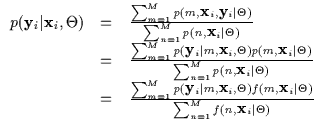(7.9)

Thus, Equation 7.8 can also be written as in Equation 7.10 in the experts-gates formalism. This formalism indicates that the parameters in the experts can often be re-written independently of the parameters of the gates. In other words, the conditional densities and the marginal densities (in appropriate coordinates) can be expressed with separate degrees of freedom. This allows us to decouple the conditional likelihood maximization further. The function Q here is a general bound for any conditional density. Thus, for the CEM algorithm, the computation of the Q function here is effectively the CE or Conditional Expectation step. The M-step, or Maximization, is specific to the density we are currently considering.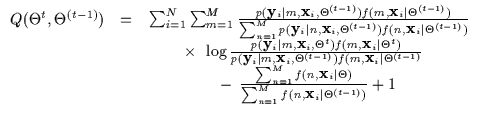(7.10)

Although we are bounding conditional densities, note that we are also abiding by the convention that the marginal densities can be expressed as functions f since the integration to 1 over the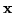domain is not a requisite for conditional densities. Of course, we can, at any time, re-normalize the gates and express proper joint densities. Equation 7.11 depicts a more practical expression for the Q function where the computations of the CE step are completed and are used numerically. The M-step then involves taking derivatives of the Q function or applying further bounds to maximize the current Q. We consider a specific cases where the densities come from a particular family of models (i.e. conditioned Gaussian mixture models ) and see how the bounding can be applied further. This will be the M-step for the Gaussian mixture case. Similar derivations can be performed for other families.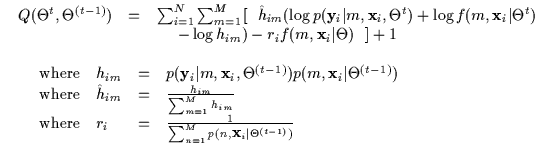(7.11)Next: Continuous Hidden Variables CEM Up: From EM to CEM Previous: From EM to CEM
Tony Jebara
1999-09-15